## Alternate formatsTrades math workbook[PDF - 1.08 MB]

Large print, braille, MP3 (audio), e-text and DAISY formats are available on demand by ordering online or calling 1 800 O-Canada (1-800-622-6232). If you use a teletypewriter (TTY), call 1-800-926-9105.

## Introduction

• discover how journeypersons use numeracy on the job
• find out which numeracy skills you may need to improve

The workbook is divided into four sections, each of which highlights different numeracy skills that journeypersons use on the job.

1. Measurement and calculation:

Skills used to measure and describe the physical world, for example by taking measurements and calculating area and volume.
2. Money math:

Skills used in paying and receiving money on the job, for example in handling cash, making change, preparing bills or making payments.
3. Scheduling, budgeting and accounting:

Skills used to manage time and money, for example in planning and keeping track of how you use your time and money, in choosing the products or services that offer the best value and in using your time and money wisely.
4. Data analysis:

Skills used to solve problems by analyzing and comparing numerical data.

An answer key is provided at the end of the workbook to help you review your answers and find out which areas of numeracy you may need to improve.

## Math foundations

Trades occupations require all or most of the math foundations listed below.
Math foundation skills Workplace examples
Whole numbers

for example: 3, 14
Read, write, count, round off, add, subtract, multiply and divide whole numbers.
• Order supplies.
• Take stock inventory.
• Count parts.
Integers

for example: -5, 0, 11
• Use survey tools.
• Set up computer numerical control programs.
• Measure air pressure.
Fractions

for example: 1/8", 1/4"
Read, write, round off, add, subtract, multiply or divide fractions. Multiply or divide by a fraction.
• Take and record imperial measurements.
• Determine tool or material sizes.
• Calculate quantities.
Decimals

for example: 8.50, 0.00375
Read, write, round off, add or subtract, multiply or divide decimals. Multiply or divide by a decimal.
• Handle money.
• Take and record metric measurements.
• Measure tolerances.
• Select tool sizes.
Percentages

for example: 10%, 42%
Read and write percentages. Calculate the percentage one number is of another. Calculate a percentage of a number.
• Calculate tax.
• Describe in terms of a proportion of maximum capacity or an amount of progress towards completion.
Equivalent numbers

for example: 1/2 = 0.5 = 50%
Convert between fractions, decimals and percentages.
• Convert decimal readings on gauges to percent of output.
• Convert decimals to fractions to select the correct part or size of tool.
• Convert quantities of ingredients to decimals to calculate cost.
Other real numbers

for example: v36, 92, 2.2 x 103, p
Use square roots, powers, scientific notation and significant digits.
• Calculate power and current in three-phase motors.
• Use square roots to calculate dimensions for a staircase.
• Use powers to express the volume of tanks.
Equations and formulas Solve problems using equations with one unknown quantity. Use formulas by inserting quantities. Solve quadratic equations.
• Determine where to place holes.
• Calculate the correct angles for rigging loads.
• Set food prices.
• Use Ohm's law to check motor voltage.
Rates, ratios and proportions Use a rate comparing 2 quantities with different units. Use a ratio comparing 2 quantities with the same units. Use a proportion comparing 2 ratios or rates.
• Adjust ingredients in a recipe to make more servings.
• Calculate speed and feed rates of a machine.
• Calculate airflow rates.
Measurement conversions Convert between imperial and metric (SI) measurements. Convert to another unit within a measurement system.
• Convert units to select wrench sizes.
• Cut lengths of wire.
• Mix colouring agents.
• Meet product specifications.
• Calculate airflow.
• Use scale drawings.
Areas, perimeters and volumes Calculate areas, perimeters and volumes.
• Calculate the area or perimeter of a work surface to be painted, sodded or caulked.
• Calculate the volume of gasoline additives or concrete required.
• Calculate the capacity of a storage tank.
Geometry Apply geometric concepts such as parallelism, perpendicularity and tangents.
• Find the centre of a room to install fixtures.
• Cut hair using angles.
• Cut slopes to fabricate ramps.
• Use angles to lay out patterns for materials.
Trigonometry Use trigonometry to determine the size of an unknown side or angle of a triangle.
• Calculate angles for a circular staircase.
• Place holes on a part.
• Make bolt patterns for drilling or machine installation.
• Find offsets.
Summary calculations Calculate averages and rates other than percentages, proportions or ratios. Calculate averages for:
• fuel or power consumption;
• tool lifespan;
• speed and feed rates;
• material production; and
• time needed to perform tasks.
Statistics and probability Use statistics and probability to draw conclusions.
• Estimate how much of something clients use.
• Predict sales trends.
• Determine the probability of equipment and parts failure.
• Describe the progress of fabrication and installation tasks.

## Section 1: Measurement and calculation

Measurement is the way that numbers are used most often in the trades. Measurement and calculation are used to measure and describe the physical world, for example by taking measurements and calculating area and volume. The following are 3 typical workplace examples of measurement and calculation:

• Construction electricians take measurements and perform calculations to make sure that electrical installations meet electrical code requirements.
• Carpenters take precise measurements using survey equipment.
• Plumbers perform calculations using trigonometry to design, fabricate and install pipe that needs to go around an obstacle.

### Using measuring tools

Journeypersons who build things in their work use measuring tapes, laser survey equipment, micrometers, scales and other tools to measure on a daily basis. They work with both imperial and metric measurements on the job.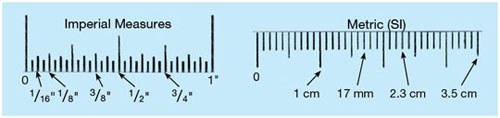1. Enter the correct length beside each arrow on the measuring tape. Remember to include the correct unit (inches or centimetres). 2 examples are provided for you.• Answer:Math foundation skills: Whole numbers, fractions, decimals (imperial, metric)

2. Label the following measurements (a–f) on the measuring tape with an arrow and the letter of the question. 2 examples have been done for you.

x. 37 1/2" y. 1.01m

a.39 1/8 in.

b.3 ft. 4 3/4 in.

c. 3.5 ft.

d. 93 cm

e. 0.992 m

f. 107.5 cm• Answer: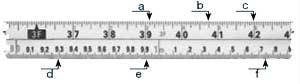Math foundation skills: Whole numbers, fractions, decimals (imperial, metric)

3. Carpenters take readings of elevations, directions and lengths of property lines using a levelling rod. The red numbers on the rod represent the number of feet, and the black numbers represent tenths of a foot.

Record the readings below. The first one has been done for you.

Note: The red numbers are larger than the black numbers when they mark an even number of feet (for example, 3.0 feet), but smaller when they appear as “reminders” in between.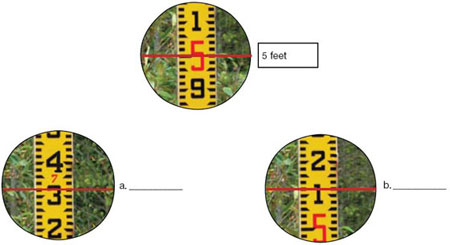Question 3a:

• 7.32 feet
• Math foundation skills: Whole numbers, decimals

Question 3b:

• 5.12 feet

Math foundation skills: Whole numbers, decimals

### Using formulas

The formulas in the box below will help you solve the workplace problems in this section.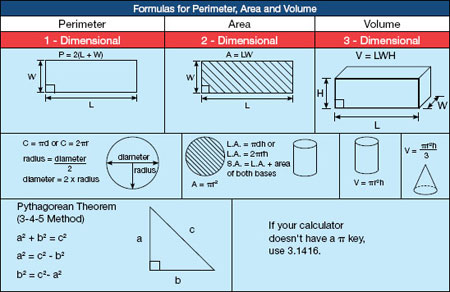Whether you are in a classroom or on the job, it is helpful to develop your own method for solving math problems involving measurements. The steps below can help you do this.

Steps:

1. Draw a diagram and label it with measurements
• Identify the information you need.
• Think about the units you are working with (for example, square metres, loads, feet).
2. Decide which formula or formulas you will need to use.

Note: Use your calculator to complete the questions in this workbook.

When you’re on the job, using a calculator saves time. Become familiar with your calculator so that you can use all of its features.
1. A carpenter is building a temporary fence around a building site. How many metres of fencing are required for a site that is 47.8 m × 30.3 m? Round your answer up to the nearest metre.

Use the problem-solving steps:

1. Draw
2. Decide
3. Calculate

2 (47.8 m + 30.3 m) = 156.2 = 157 m

Math foundation skills: Perimeters (metrics)

2. How many studs will a carpenter need to frame the exterior walls of the building below? Use the formula given below to estimate the number of studs52' + 14' + (52' – 30' 8") + (28' – 14 ‘) +

30' 8" + 28' = 160'

160 ÷ 2 = 80 studs

Math foundation skills: Perimeters (imperial)

3. How many 3.7 L containers of bonding product does a floorcovering installer need to install vinyl sheet flooring in a room measuring 9.2 m × 7.8 m?

Only full containers can be ordered.

Typical recommended trowel and approximate coverage.
Typical recommended trowel

(depth, width, space)
Approximate coverage Trowel
Fibrous felt-backed vinyl sheet goods:

1/32" × 1/16" × 1/32"

(0.8 mm × 1.6 mm × 0.8 mm)
185–245 sq. ft./U.S. gal.

(4 5–6 0 m2/L)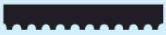9.2 m × 7.8 m = 71.76 m2

71.76 m2 ÷ 4.5 m2/L = 15.95 L

15.95 L ÷ 3.7 L = 4.31 = 5 containers

Math foundation skills: Area (metrics)

4. A bricklayer is covering a playground surface with rubber tiles. Each 2-ft 2 tile costs \$13.29. Calculate how much it will cost to cover the playground.

Note: Only whole tiles can be ordered, so you will need to round your answer up when you calculate how many tiles are needed.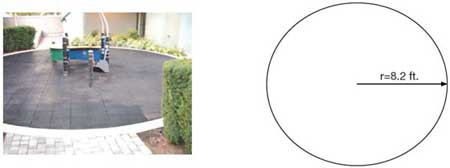3.1416 (8.2 ft. × 8.2 ft.) = 211.24 ft.2

(211.24 ft.2 ÷ 2 ft. 2/tile) = 105.62 = 106 tiles

106 tiles × \$13.29/tile = \$1,408.74

Math foundation skills: Area (imperial)

5. A landscape horticulturalist needs to order enough sand to create a border 152 mm deep around a square surface, as shown below. How many cubic metres of sand are needed?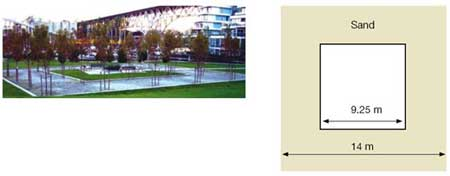(14 m)2 – (9.25 m)2 = 110.44 m2

110.44 m2 × 0.152 m = 16 79 m3

Math foundation skills: Volume (metrics)

6. A construction craft worker needs to know how much material is in the cone-shaped pile shown below. Calculate the approximate volume of the pile in cubic yards.

Use this formula to calculate the radius of a pile of material:

r = ¾ × height

27 ft. 3 = 1 yd. 3r = ¾ × 16 ft. = 12 ft.

(3.1416 × (12 ft.)2 × 16 ft.) ÷ 3 = 2 412.75 ft. 3

2 412.75 ft. 3 ÷ 27 ft. 3/yd 3 = 89.36 yd 3

Math foundation skills: (Volume imperial)

7. Heat and frost insulators cover pipes to keep substances hot or cold. How many square metres of material are needed to insulate a pipe that is 6 m long and has a diameter of 2 m?

Think of the cylinder as being laid out flat so that the circumference becomes the width measurement.

Use this formula: pdh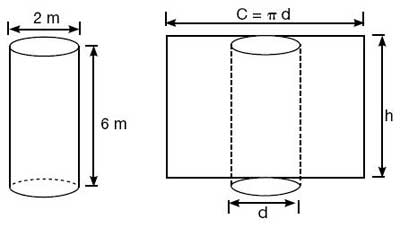3.1416 × 2 m × 6 m = 37.6992 = 37.7 m2

Math foundation skills: Area (metrics)

8. Electricians calculate the total resistance of parallel electrical circuits. Use the formula below to calculate the total resistance for the circuit shown.8 ohms ÷ 4 resistors = 2 ohms

Math foundation skills: Equations and formulas

9. Plumbers fit pieces of pipe for custom jobs. What is the centre-to-centre (c–c) length of the pipe shown below?222 + 182 = 808

v808 = 28.43 cm

Math foundation skills: Trigonometry

10. Carpenters perform calculations to help them lay out and construct wooden stairs. Calculate the length of the stringer (c) for the stairs shown below.1 0002 + 1 2552 = 2 575 025

v2 575 025 = 1 605 mm

Math foundation skills: Trigonometry

11. Refrigeration and air conditioning mechanics and sheet metal workers build and install transition elbows to connect different sizes of ducts. Calculate the length of the diagonal side of the transition elbow shown below.152 + (12– 8)2 = 241

v241 = 15.52 inches

Math foundation skills: Trigonometry

12. Plumbers calculate water pressure in pounds per square inch (psi). What is the water pressure for a 28-foot vertical pipe full of water?

Use this formula:

Water pressure (psi) = h × 0.433

28 × 0.433 = 12.12 psi

Math foundation skills: Equations and formulas

13. Sheet metal workers install ducts in buildings.

The equation below shows the relationship between:

• airflow volume in cubic feet per minute (V);
• air velocity in feet per minute (v); and
• area of a cross-section of duct in square feet (A).

V = A × v

a. Calculate the area in square feet of a cross-section of an 8 in. × 24 in. duct.

1 ft. 2 = 144 in. 2

b. Calculate the airflow volume if the air velocity in the same duct is 1,200 feet per minute.

Question 13a:

• 8 in. × 24 in. = 192 in. 2
• 192 in. 2 ÷ 144 in. 2/ft. 2 = 1.33 ft 2
• Math foundation skills: Area (imperial)

Question 13b:

• 1.33 ft. 2 ×1 200 ft. /min = 1 596 ft. 3/min
• Math foundation skills: Equations and formulas

### Using drawings

Journeypersons working on a construction site follow specifications from a set of drawings or prints that show different views of the finished building project. Journeypersons in all trades scan the drawings for the detailed information they need.

Journeypersons often convert inches to fractions or decimals of a foot.

3 in = ¼ ft. or 0.25 ft.

6 in = ½ ft. or 0.5 ft.

9 in = ¾ ft. or 0.75 ft.

12 in = 1.0 ft.

Adding and subtracting feet and inches:

Step 1: Line up the measurements so that like units are under like units.

Step 2: Add or subtract the inches. Add or subtract the feet.

Step 3: Change the inches to feet (divide by 12).

Example:

Step 1
7 ft. 11 in.
+ 2 ft. 9 in.
___________

Step 2
7 ft. 11 in.
+ 2 ft. 9 in.
___________
9 ft. 20 in.

Step 3
20 in. = 1 ft. 8 in.

Step 4
9 ft.
+ 1 ft. 8 in.
___________
10 ft. 8 in.

Look at the drawings for a residence to complete the questions below.

1. The walk-in closet measures 1¼ in. × 1½ in. on the drawing. What are the actual dimensions of the closet?

5/4 × 1 ft. = 5 ft.

3/2 × 1 ft. = 6 ft.

Dimensions = 5 ft. × 6 ft.

Math foundation skills: Rates, ratios and proportions

2. How many pocket (sliding) doors are needed?3

Math foundation skills: Whole numbers

3. What is the exterior length from A to B?

2' 2" + 8' + 2' 2" = 12' 4"

Math foundation skills: Measurement conversions

4. Carpet costs \$28.50 per square yard. What is the cost of carpet for the master bedroom and closet?

1 yd.2 = 9 ft.2

A = 12 ft. ×14 ft. = 168 ft.2

168 ft.2 + 30 ft.2 = 198 ft.2

198 ft.2 ÷ 9 ft. 2/yd.2 = 22 yd.2

\$28.50/yd.2 × 22 yd.2 = \$627.00

Math foundation skills: Area, measurement conversions

5. Estimate the number of drywall sheets needed for the walls of the ensuite bathroom.

Drywall sheets: 4 ft. × 8 ft.

Height of room: 8 ft

Width of pocket door: 3 ft.Method 1:

• Height of drywall sheets = height of room
• 5 ft. + 7.67 ft. + 5 ft. + 7.67 ft. = 25.34 ft.
• 25.34 ft. – 3 ft. = 22.34 ft.
• 22.34 ft ÷ 4 ft. /sheet = 5.585 = 6 sheets

Method 2:

• 5 ft. + 7.67 ft. + 5 ft. + 7 67 ft. = 25.34 ft.
• 25.34 ft. – 3 ft. = 22.34 ft.
• 22.34 ft. × 8 ft. = 178.72 ft.2
• 4 ft. × 8 ft. = 32 ft.2
• 178.72 ft. 2 ÷ 32 ft.2 = 5.585 = 6 sheets

Mobile crane operators estimate weight loads. Calculating safe loads protects the equipment, the materials it carries, the workers on the job site and the general public.

Practice exercise

Estimate the weight of a 4 ft. × 18 in. aluminum plate 3/8 in. thick.

Aluminum plate 1/8 in. thick weighs approximately 1.75 pounds per square foot.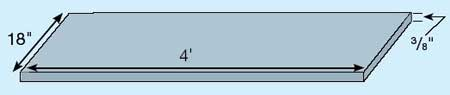Steps:

1. Draw a diagram and label it with the measurements.

2. Calculate the area.

Area = L × W

= 4 ft. × 1.5 ft.

= 6 ft.2

3. Calculate the weight.

a) Weight of 1 ft.2 of 3/8 in. aluminum plate: --> 3/8 in. ÷ 1/8 in. = 3 --> 1.75 lb./ft.2 × 3 = 5.25 lb./ft.2

b)

Area of plate
× Weight per square foot:
_____________________
= Weight in pounds

6 ft.2
× 5.25 lb./ft.2
_______________
= 31.5 lb.

The aluminum plate
weighs approximately
31.5 pounds.

1. Boilermakers and ironworkers estimate the weight of materials used in fabrication. Calculate the approximate weight of a 2 ft. × 18 ft. steel plate ¾ in thick.

1" steel plate weighs about 40 lb./ft.2.

2 ft. × 18 ft. = 36 ft.2

¾ × 40 lb. /ft.2 = 30 lb. /ft.2

36 ft.2 × 30 lb. /ft.2 = 1 080 lb.

Math foundation skills: Equations and formulas

2. A mobile crane operator estimates the weight of a concrete panel to make sure that the crane can lift it safely. Calculate the approximate weight of the panel shown below.

Reinforced concrete weighs about 150 lb./ft.3.26' × 30.17' × 0.5' = 392.21 ft.3

392.21 ft. 3 × 150 lb. /ft.3 = 58 831.5 lb.

Math foundation skills: Equations and formulas

### Working with quantities

Rates and ratios are used to compare 2 quantities. Both can be expressed in the following forms: 1 to 2, 1:2 or ½.

Rates compare 2 quantities with different units. For example, a rate can be used to describe the flow of a liquid in litres per second.

Example: 1 tablespoon of flour : 1 cup of milk

Ratios compare 2 quantities with the same units. For example, a ratio can be used to describe the number of parts of water and colouring agents to combine.

Example: 1 part water

___________

2 parts cement

Proportions compare 2 ratios or 2 rates.

1. A sewer line slopes at ¼" per foot. Calculate the total fall in 30 feet.

Use this formula:

Total fall = length × grade

Total fall = 30 ft. × ¼ in/ft. = 7.5 ft.

Math foundation skills: Rates, ratios and proportions

2. Automotive service technicians occasionally need to convert kilometres to miles for American customers. An oil change is due at 35,000 km. What is the same distance in miles?

1 km = 0.6214 mi.

35 000 km × 0.6214 mi/km = 21 749 mi.

Math foundation skills: Rates, ratios and proportions

3. A hairstylist is mixing a hair treatment. The client has long hair, so the hairstylist starts with 1¼ scoops of powder lightener.

Mixing: Measure 1 level scoop of powder lightener into a non-metallic bowl or bottle. Add 1 oz. (30 g) of the booster and 2 fl. oz. (60 mL) of conditioning creme. Mix thoroughly to achieve a creamy consistency.

a. Calculate the number of grams of booster needed.

b. Calculate the number of millilitres of conditioning creme needed.

Question 3a:

• 5/4 × 30 g = 37.5 gMath foundation skills: Rates, ratios and proportions

Question 3b:

• 5/4 × 60 mL = 75 mL

Math foundation skills: Rates, ratios and proportions

4. Cooks often change the yield of recipes to serve more or fewer customers. The following recipe makes 30 pancakes. Adapt the recipe to make 75 pancakes.

Use proportions to calculate how much of each ingredient is needed. The first ingredient is done for you using 2 different methods.

Method 1:

625 g = ? g

____ ____

30 75

625 x 75 = 46875 ÷ 30 = 1562.5 g

____

30

Method 2:

625 ÷ 30 = 20.883

75 x 20.833 = 1562.5 g

Ingredient measurements
Ingredient Amount for 30 Amount for 75
Flour 625 g 1562.5 g
Sugar 60 g
Baking powder 30 g
Eggs 4
Milk 1 L
Melted butter 125 g

amount × 75 = quantity of ingredients

___________
30

sugar – 150 g; baking powder – 75 g;

eggs – 10; milk – 2.5 L: butter 312.5 g

Math foundation skills: Rate, ratios and proportions

5. Product labels provide information about quantities to mix. Use the label to answer the questions below it.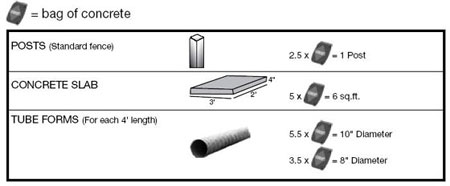1. A carpenter is building a fence with 9 posts. How many bags of concrete are required to set the posts?
2. Calculate the number of bags of concrete mix required for a slab measuring 5' × 2'4" × 6".
3. Tube forms are used to form concrete columns. The concrete is mixed at a ratio of 2.5 litres of water per bag of concrete mix. Calculate the amount of water needed for a 15-foot column with a diameter of 8 inches.

Question 5a:

• 9 posts × 2.5 bags/post = 22.5 = 23 bagsMath foundation skills: Rates, ratios and proportions

Question 5b:

• 5 bags = 3 ft. × 2 ft. × .33 ft. = 1.98 ft.3
• 5 ft. × 2.33 ft. × 0.5 ft. = 5.825 ft.3
• 5.825 ft. 3 ÷ 1.98 ft.3 = 2.94
• 2.94 × 5 bags = 14.7 = 15 bags
• Math foundation skills: Rates, ratios and proportions

Question 5c:

• 15ft. ÷ 4 ft. = 3.75
• 3.75 × 3.5 = 13.125 = 14 bags
• 14 bags × 2.5 L/bag = 35 litres of water
• Math foundation skills: Rates, ratios and proportions

## Section 2: Money math

Money math is used in paying and receiving money on the job, for example in handling cash, preparing bills or making payments. The following are 3 typical workplace examples of money math.

• Cooks use petty cash to purchase small quantities of supplies that are needed immediately.
• Hairstylists prepare bills and collect cash, debit and credit card payments for their services. They charge a set rate for each service and add applicable taxes, such as the GST.
• Automotive service technicians calculate the total cost of repair jobs including parts, labour, markup and taxes, and enter these amounts on estimates or finished work orders.

### Calculating increases and decreases

In some shops, products are bought at wholesale prices and marked up to sell to customers. Here is one way to calculate markup and sales tax.

Calculating increases
Method Examples

Markup

• 100% + markup = total %
• change % to a decimal
• total decimal × unit price = selling price

An item with a wholesale price of \$14.35 is marked up 22%. Calculate the selling price.

100% + 22% = 122%

122/100 = 1.22

1.22 × \$14.35 = 17.507 = \$17.51

Sales tax

• 100% + tax = % including tax
• change % to a decimal
• total decimal × cost = after-tax total

Calculate the after-tax cost of 2 hours of labour at a rate of \$45/hour.

2 hours × \$45.00 = \$90.00 labour cost

100% + 7% = 107%

107/100 = 1.07

1.07 × \$90.00 = 96.3 = \$96.30

Products are sometimes discounted when they are discontinued or when the supplier has a promotion. Here is one way to calculate a discount.

Calculating discounts
Method Examples
• 100% – % discount = new %
• change % to a decimal
• decimal × unit price = sale price

An item with a regular price of \$10.89 is discounted 15% Calculate the sale price.

100% – 15% = 85%

85/100 = 0.85

0.85 × \$10.89 = 9.257 = \$9.26

1. In a hair salon, products are marked up for resale. Calculate the selling prices of the items listed below.

Calculate the selling prices of the items
Wholesale price Markup Selling price
a. \$97.25 10%
b. \$249.99 15%
c. \$6.50 8%

Question 1a:

• (1.00 + 10/100) × \$97.25 = \$106.98
• Math foundations skills: Percentages

Question 1b:

• 1.15 × \$249.99 = \$287.49
• Math foundations skills: Percentages

Question 1c:

• 1.08 × \$6.50 = \$7.02
• Math foundations skills: Percentages
2. Calculate the after-tax cost of the items listed below.

Calculate the after-tax cost of the items
Cost Tax Total
a. \$73.50 12%
b. \$1,847.00 13%
c. \$86.75 8%

Question 2a:

• 1.12 × \$73.50 = \$82.32
• Math foundation skills: Percentages

Question 2b:

• 1.13 × \$1,847 = \$2,087.11
• Math foundation skills: Percentages

Question 2c:

• 1.08 × \$86.75 = \$93.69
• Math foundation skills: Percentages
3. In a hair salon, products are discounted for promotions. Calculate the sale prices of the items listed below.

Calculate the sale prices of the items
Price Discount Sale price
a. \$85.40 10%
b. \$1,348.00 33%
c. \$459.75 40%

Question 3a:

• (1.00 – 10/100) × \$85.40 = \$76.86
• Math foundation skills: Percentages

Question 3b:

• 0.67 × \$1,348 = \$903.16
• Math foundation skills: Percentages

Question 3c:

• 0.60 × \$459.75 = \$275.85
• Math foundation skills: Percentages

### Invoicing for services

Journeypersons who install, maintain and repair equipment often make invoices for services and are paid in cash, by cheque or by credit card on the job. Calculating tax is a typical task in these situations.

1. a) Complete the invoice on the next page for the following services:

• Repair dishwasher
• Model #MDB7601AWW
• Clean pump assembly, Test OK
• Labour 1.2 hours at \$88.25 per hour (5% GST)
• Parts \$2.80 (5% GST + 6% PST)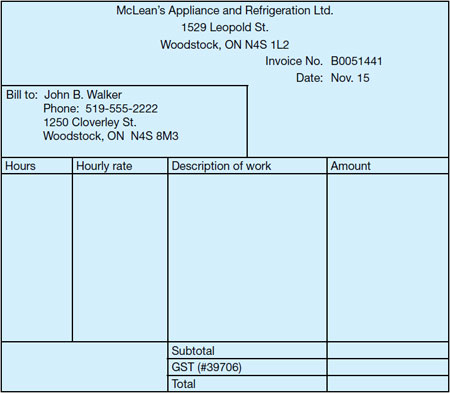b) The customer pays the bill in cash using the following amounts:

1 × \$100

1 × \$20

1 cent

How much change should the technician give the customer? Enter the amounts of money that could be given as change in the table below. (There are several correct answers.)

Change to be given
\$50 \$20 \$10 \$5 \$2 \$1 25¢ 10¢

Question 1a:

• \$105.90 × 1.05 = \$111.20 (labour)
• \$2.80 × 1.11 = \$3.11 (parts)
• \$111.20 + \$3.11 = \$114.31
• Math foundation skills: Decimals

Question 1b:

Change: \$120.01 – \$114.31 = \$5.70

\$50 \$20 \$10 \$5 \$2 \$1 25¢ 10¢
1 2 2

Math foundation skills: Decimals

## Section 3: Scheduling, budgeting and accounting

Scheduling, budgeting and accounting are used to manage time and money, for example in planning and keeping track of how you use your time and money, in choosing the products or services that offer the best value and in using your time and money wisely. The following are 3 typical workplace examples of scheduling, budgeting and accounting.

• Industrial mechanics (millwrights) schedule tasks for construction, repair and maintenance projects. They also create maintenance schedules for equipment in manufacturing plants.
• Machinists adjust daily work schedules to accommodate rush jobs or jobs that take longer than estimated. For example, they may change machining processes or the order of jobs. They consider whether others are affected by changes in the workflow and try to minimize the disruption.
• Cooks establish weekly budgets that include the cost of fresh food, shelf items and kitchen staff requirements. They also establish separate budgets for each of the catering events for the week. The costs included in the catering budgets vary depending on the menu items, number of courses and number of people served.

### Comparing values

Use a table structure to compare items.

Table to compare items
Points of comparison Item 1 Item 2 Item 3
Cost 1
Cost 2
Cost 3

The problems in this section require you to locate and understand information in documents.

Coating products can be applied by either a trowel-on or a roll-on method.

1. A concrete finisher estimates the cost of resurfacing the sidewalk pictured using 2 different methods for placing concrete. The trowel-on method lasts longer but costs more.

Estimate the cost difference given:

• The concrete finisher charges \$37.50 per hour;
• Each coat takes approximately 1 hour and 30 minutes to apply.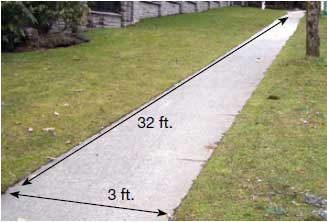Roll-on method
Component 9.29 m2

(100 sq. ft.)
18.58 m2

(200 sq. ft.)
27.87 m2

(300 sq. ft.)
37.16 m2

(400 sq. ft.)
No primer required
Step 1: Roll-on

stone coat

\$89.00/ 9.2 L
1 × 9.2 L 2 × 9.2 L 3 × 9.2 L 4 × 9.2 L
Step 2: Protective

top coat

\$26.78/946 mL
1 × 946 mL 2 × 946 mL 1 × 3.78 L 1 × 3.78 L
Trowel-on method
Component 9.29 m2

(100 sq. ft. )
18.58 m2

(200 sq. ft. )
27.87 m2

(300 sq. ft. )
37.16 m2

(400 sq. ft. )
Step 1:

Primer coat

\$48.20/3.78 L
1 × 3.78 L 1 × 3.78 L

+

1 × 946 mL
2 × 3.78 L 3 × 3.78 L
Step 2: Trowel-on

stone coat

\$115.07/15.1 L

\$53.25/3.78 L
1 × 15.1 L

+

2 × 3.78 L
3 × 15.1 L 4 × 15.1 L

+

2 × 3.78
6 × 15.1 L
Step 3: Protective

top coat

\$26.78/946 mL
1 × 946 mL 2 × 946 mL 1 × 3.78 L 1 × 3.78 L

Area of sidewalk = 32 ft. × 3 ft. = 96 ft.2

Roll-on: (1 5 h × 2) × \$37.50/h = \$112.50

\$89.00 + \$26.78 = \$115.78

\$112.50 + \$115.78 = \$228.28

Trowel-on: (1.5 h × 3) × \$37.50 = \$168.75

\$48.02 + \$115.07 + (2 × \$53.25) + \$26.78 = \$296.55

\$168.75 + \$296.55 = \$465.30

\$465.30 – \$228.28 = \$237.02

The trowel-on method costs about \$240.00 more

Math foundation skills: Decimals

### Accounting for cost

Many journeypersons give estimates to customers. This involves telling the customer approximately how much he or she should expect to pay for a certain item.

In the example below, a journeyperson estimates that a sink will cost \$100. If the sink is not purchased or costs less than \$100, the savings are shown in brackets. This means the actual cost is less than budgeted.

Example of savings shown in brackets
Item Allowance (\$) Actual (\$) Difference +/- (\$)
Sink 100.00 75.99 (24.01)

If the sink costs more than \$100, the extra cost is shown without brackets. This means the actual cost is more than budgeted.

Example of extra cost shown without brackets
Item Allowance (\$) Actual (\$) Difference +/- (\$)
Sink 100.00 123.67 23.67
1. Journeypersons compare estimates with actual costs. When the customer selects the specific item they want to purchase, the journeyperson can point out how much higher or lower than estimated the actual cost will be.

Use the renovation invoice below to answer the following questions.

1. Compare the allowance for the floor coverings with the actual cost. Enter the difference on the invoice.

2. A contractor is bidding on apartment renovations and needs to submit an estimate for installing the same bathroom mirror, wall tile and toilet in 5 units. Use the actual costs from the invoice to calculate the estimate the contractor should provide. Add 10% to account for rising costs.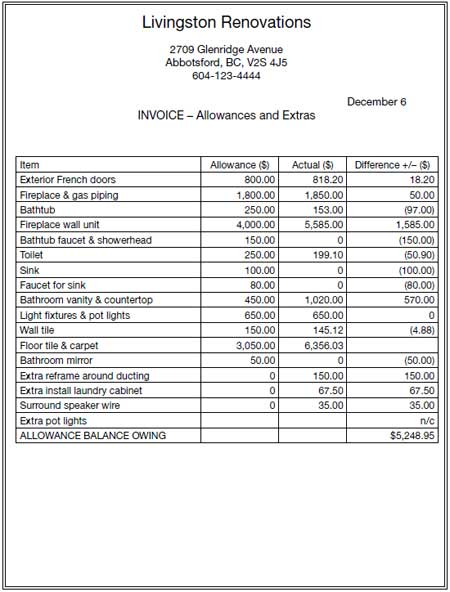Question 1a:

• \$3,050 – \$6,356.03 = –\$3,306.03
• Entry on invoice: (3,306.03)
• Math foundation skills: Integers

Question 1b:

• (\$153.00 + \$145.12 + \$199.10) × 5 = \$2,486.10
• 1.10 × \$2,486.10 = \$2,734.71
• Math foundation skills: Integers

## Section 4: Data Analysis

Data analysis is used to solve problems by analyzing and comparing data. The need for these skills is increasing as computer programs make data more available. The following are three typical workplace examples of data analysis.

• Automotive service technicians analyze readings from tests of vehicle electrical systems to diagnose problems such as an engine that will not start.
• Machinists review quality control data to examine trends in machine performance. For example, they may compare the finished dimensions of parts produced at different points in the machining cycle to decide when to replace tooling or recalibrate machinery.
• Construction electricians use the results of electrical measurements (e g, draw, voltage, torque and temperature) taken at several points in a circuit to analyze circuit operation, to troubleshoot electrical problems and to increase electrical efficiency.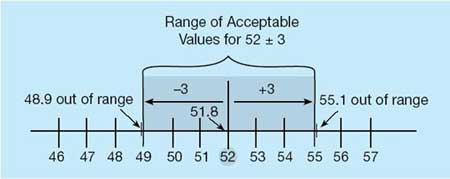### Calculating tolerances

1. Sheet metal workers, machinists, refrigeration and air conditioning mechanics work to very tight tolerances. Decide if the measures in the following table are within specifications. If they are, place a v in the table; if they are not acceptable, place an X.

± means plus or minus

Table showing if measures are within specification
Specification Measure v/X
a. 22.5° ± 2° 20.1°
b. 0.850 m ± 0.020 m 0.827 m
c. 0.750 L ± 0.015 L 0.761 L

Question 1a:

• X not acceptable
• 22.5° – 2° = 20.5°
• 20.1° is not between 20.5°
• and 22.5° = not acceptable
• Math skills foundation: Integers

Question 1b:

• X not acceptable
• 0.850 m – 0.020 m = 0.830 m
• 0.827 m is not between 0.830 m and 0.850 m = not acceptable
• Math skills foundation: Integers

Question 1c:

• ✓acceptable
• 0.750 L + 0.015 L = 0.765 L
• 0.761 L is between 0.750 L and 0.765 L =
• acceptable
• Math skills foundation: Integers
2. An ironworker rigging a load plans to use a ¾-inch wire-rope choker hitch to lift a beam that weighs 4.6 tons. Use the table below to make a recommendation for a safer lift. (There are several correct answers.)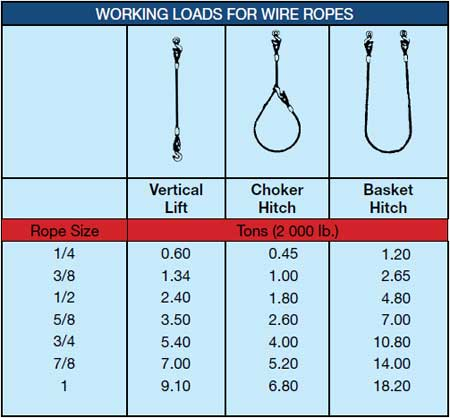Note: This capacity table is for this workbook only. On the job you should use the manufacturer's table.

• use a 3/4" rope and a vertical lift (5.4 tons max )
• use a 7/8" rope and a choker hitch (5.2 tons max )

Math foundation skills: Integers

### Using numbers in patterns

1. Automotive service technicians use diagnostic equipment to analyze problems in exhaust systems. When the system is operating correctly, the Y or vertical axis on the graph should show signals that constantly change from under 0.2 volts to over 0.8 volts.

The graph below shows 2 sets of numerical data. The top set shows the reading from the driver’s side of the engine and the bottom set shows the reading from the passenger’s side.

How are the 2 sets of graph lines different?